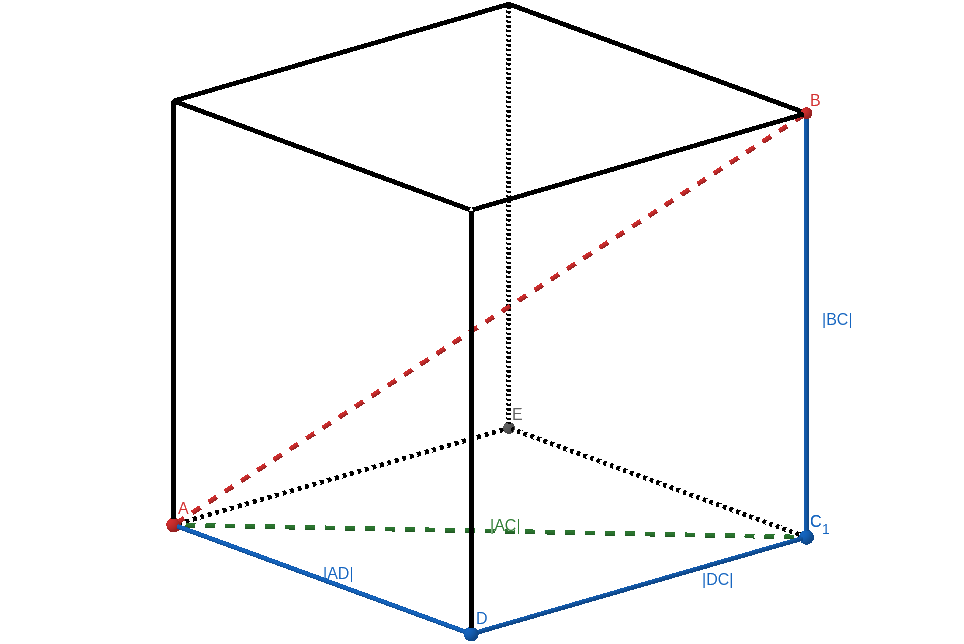# 距离

## 欧氏距离

### 二维空间

#### 定义#### 解释### n 维空间

#### 引入#### 定义#### 解释

NOIP2017 提高组 奶酪 就运用了这一知识，可以作为欧氏距离的例题。## 曼哈顿距离

### 定义### 解释间，黄线、橙线都表示曼哈顿距离，而红线、蓝线表示等价的曼哈顿距离，绿线表示欧氏距离。### 性质

• 非负性

曼哈顿距离是一个非负数。• 统一性

点到自身的曼哈顿距离为• 对称性的曼哈顿距离相等，且是对称函数。• 三角不等式

从点的直接距离不会大于途经的任何其它点的距离。### 例题

P5098「USACO04OPEN」Cave Cows 3

••1 2 3 4 5 6 7 8 9 10 11 12 13 14 #include using namespace std; int main() { int n, x, y, minx = 0x7fffffff, maxx = 0, miny = 0x7fffffff, maxy = 0; scanf("%d", &n); for (int i = 1; i <= n; i++) { scanf("%d%d", &x, &y); minx = min(minx, x + y), maxx = max(maxx, x + y); miny = min(miny, x - y), maxy = max(maxy, x - y); } printf("%d\n", max(maxx - minx, maxy - miny)); return 0; } 
 1 2 3 4 5 6 7 minx = 0x7fffffff; maxx = 0; miny = 0x7fffffff; maxy = 0 n = int(input()) for i in range(1, n + 1): x, y = map(lambda x:int(x), input().split()) minx = min(minx, x + y); maxx = max(maxx, x + y) miny = min(miny, x - y); maxy = max(maxy, x - y) print(max(maxx - minx, maxy - miny)) 

## 切比雪夫距离

### 定义维空间中切比雪夫距离的距离公式可以表示为：### 解释## 曼哈顿距离与切比雪夫距离的相互转化

### 过程个正方形是相似图形。

### 证明### 结论

• 曼哈顿坐标系是通过切比雪夫坐标系旋转后，再缩小到原来的一半得到的。
• 将一个点的坐标变为后，原坐标系中的曼哈顿距离等于新坐标系中的切比雪夫距离。
• 将一个点的坐标变为后，原坐标系中的切比雪夫距离等于新坐标系中的曼哈顿距离。

### 例题

P4648「IOI2007」pairs 动物对数（曼哈顿距离转切比雪夫距离）

P3964「TJOI2013」松鼠聚会（切比雪夫距离转曼哈顿距离）

  1 2 3 4 5 6 7 8 9 10 11 12 13 14 15 #include using namespace std; int main() { int n, x, y, a, b, minx = 0x7fffffff, maxx = 0, miny = 0x7fffffff, maxy = 0; scanf("%d", &n); for (int i = 1; i <= n; i++) { scanf("%d%d", &a, &b); x = a + b, y = a - b; minx = min(minx, x), maxx = max(maxx, x); miny = min(miny, y), maxy = max(maxy, y); } printf("%d\n", max(maxx - minx, maxy - miny)); return 0; } 
 1 2 3 4 5 6 7 8 minx = 0x7fffffff; maxx = 0; miny = 0x7fffffff; maxy = 0 n = int(input()) for i in range(1, n + 1): a, b = map(lambda x:int(x), input().split()) x = a + b; y = a - b minx = min(minx, x); maxx = max(maxx, x) miny = min(miny, y); maxy = max(maxy, y) print(max(maxx - minx, maxy - miny)) 

##距离